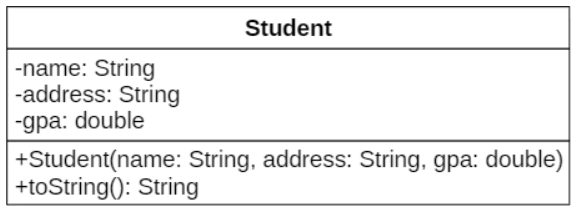# Lớp Object trong Java | Codelearn

•TuanLQ7
• Easy
• 100 Points

Create the `Student` class with the following information:Explanation:

• `name, address, gpa` attributes describe the name, address and GPA of a `Student`.
• `Student(name: String, address: String, gpa: double)` is a. constructor with parameter which is used to initializing attributes.
• `toString()` is the method which overrides `Object` class. It returns the object information in the `String` format. For example, if `name = "Kien", address = "Ha Noi", gpa = "6.6"`, the `toString()` method will return the following result: `"Name: Kien, address: Ha Noi, GPA: 6.6"`.

A program to test `Student` class:

``````public class Entry {
public static void main(String[] args) {
Student s = new Student("Kien", "Ha Noi", 6.6);
System.out.println(s);
}
}``````

When the above code is compiled and executed, it produces the following result:

``Name: Kien, addres: Ha Noi, GPA: 6.6``

### Theory

This article will give you better understanding about `Object` class. First of all, see the following example:

``````class Student{
}

public class Entry {
public static void main(String[] args) {
Student s = new Student();
System.out.println(s.hashCode());
System.out.println(s.toString());
System.out.println(s.getClass());
}
}
``````

When the above code is compiled and executed, it produces the following result:

``````1554547125
[email protected]
class OOP.Student
``````

Looking at the above example, you will wonder why the Student class has methods like `hashCode(), toString(), getClass()` when you do not declare these methods. The reason is that all classes in Java are inherited from `Object` class and `Object` class have these methods

In addition to the above methods, the `Object` class also has a number of other methods, in which `toString()` is the method used to represent the object as a string. In fact, the `toString()` is very useful. For example, when you call the `System.out.println()` function with an object, the program will display the `toString()` method of that object:

``````public class Student {
private String name;
private double gpa;

public Student(String name, String address, double gpa) {
this.name = name;
this.gpa = gpa;
}

@Override
public String toString() {
return "Name: " + name + ", address: " + address + ", GPA: " + gpa;
}
}``````

When the above code is compiled and executed, it produces the following result:

``Name: Kien, age: 23``

As you can see, it is possible to use the `toString()` method instead of the `display()` method you learned in previous articles.

### Instruction

You need to create the `Student` class like this:

``````public class Student {
private String name;